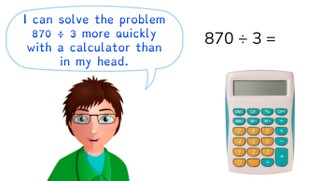Multiplying and dividing with the calculator

# Multiplying and dividing with the calculator

Students learn to multiply and divide with a calculator.

No account needed.8,000 schools use Gynzy92,000 teachers use Gynzy1,600,000 students use Gynzy

## General

The students learn to multiply and divide numbers to 10,000 with a calculator.

## Standards

CCSS.Math.Practice.MP5

## Learning objective

Students will be able to multiply and divide numbers to 10,000 using a calculator.

## Introduction

Click on the speaker to have each number read aloud. Then have the students write down each number that they hear.

## Instruction

You can have the students solve the problems on a calculator during this lesson. Show how you type the problem 17 × 35 into the calculator. Repeat this for the division problem 888 ÷ 37. After this, the students can solve a few problems on their own. Then show another group of problems, and ask the students which problems they would solve with and without using the calculator. Also ask why. Show that numbers above 1,000 get a comma on the calculator. So it is important to notice whether a mark is a comma or a decimal point. Practice this with the next few problems. Walk through the tips for using a calculator with the students. Briefly remind students of the rules for rounding numbers. Next you show how you round numbers to 2 decimal places, 1 decimal place, and to the nearest whole numbers. Now have the students solve the following problems on their own. Finally, practice solving problems in the form of a story problem.

Check whether the students can multiply and divide numbers to 10,000 with the calculator by asking the following question:
- Why is it important to be able to multiply and divide with a calculator?

## Quiz

The students test their understanding of multiplying and dividing with the calculator through ten exercises. In each exercise, they must solve a division problem using the calculator. Some of the answers must be rounded, and some of the exercises are in the form of a story problem.

## Closing

Discuss once again the importance of being able to multiply and divide numbers to 10,000 with the calculator. As a closing activity you can have the students work in pairs. Each student comes up with a number to 500. Next they multiply their number with their partner's number. They check their answers together. After this you have the students solve the problems on the interactive whiteboard, and then spin the wheel to determine if the answer should be rounded to 3 decimal places, 2 decimal places, of 1 decimal place. You can fill in these answers above the dotted lines.

## Teaching tips

Have students that have difficulty with multiplying and dividing numbers to 10,000 with the calculator first practice with typing numbers correctly into the calculator. Make sure they are using the correct digits. Also practice rounding (decimal) numbers.

## Instruction materials

Calculators

### The online teaching platform for interactive whiteboards and displays in schools

• Save time building lessons

• Manage the classroom more efficiently

• Increase student engagement